# Persamaan Differensial Biasa 2014

6 Oct 2014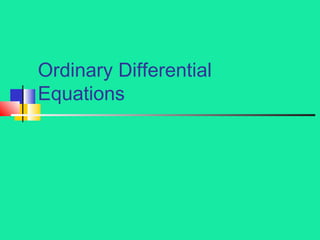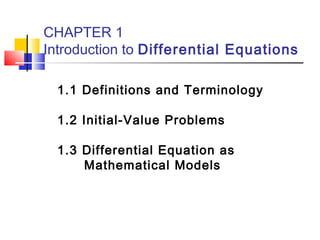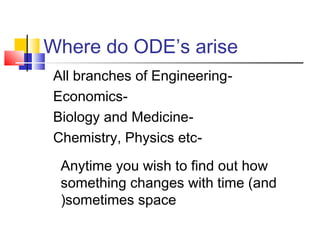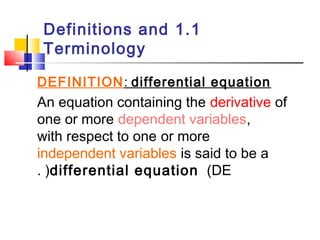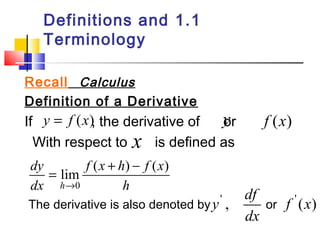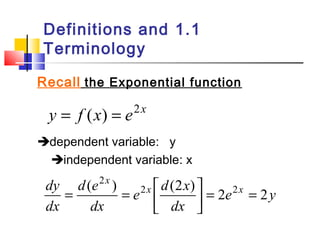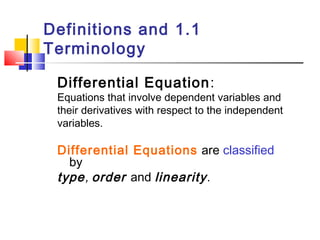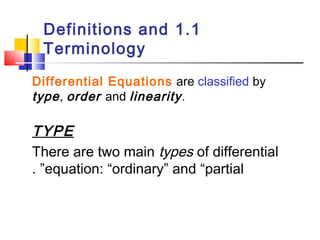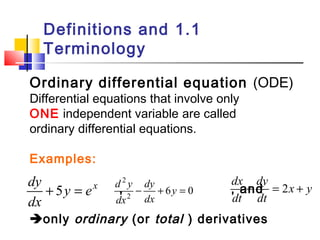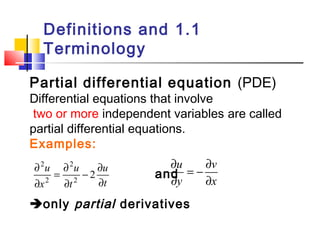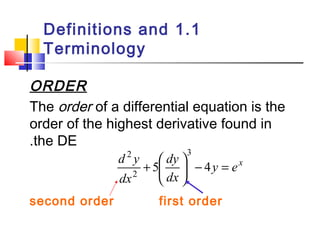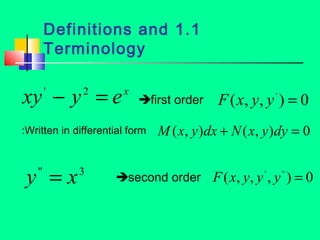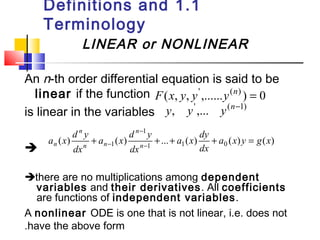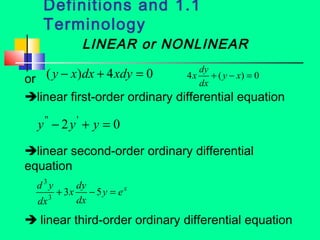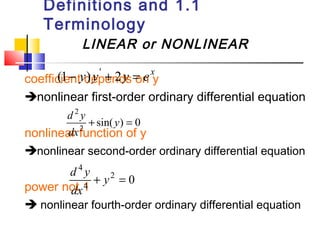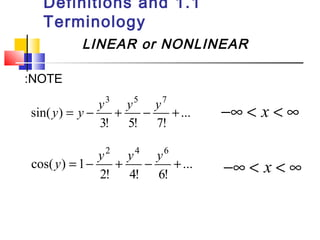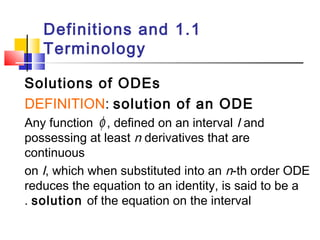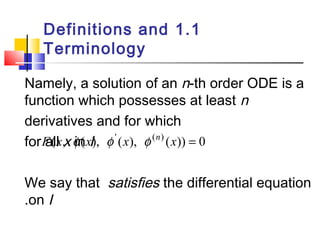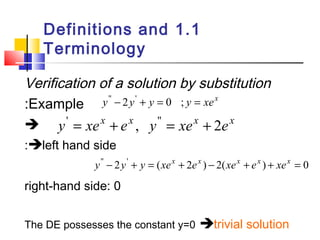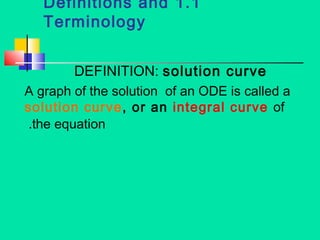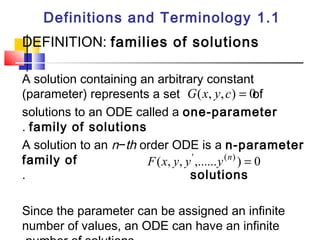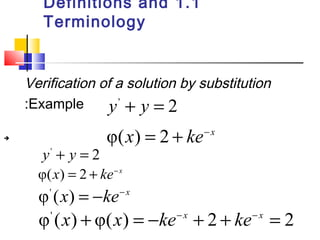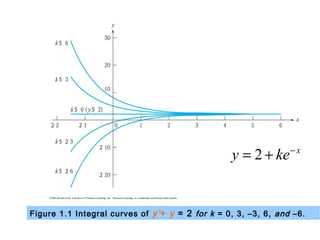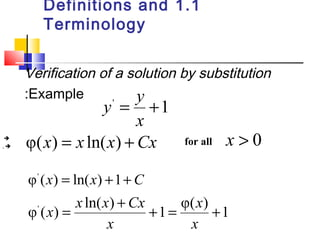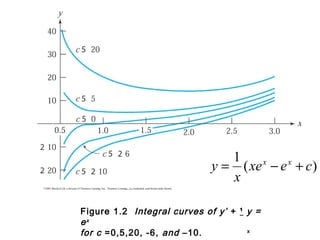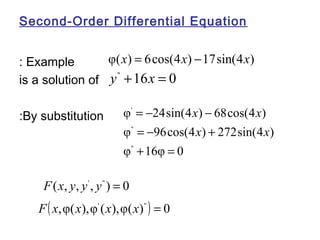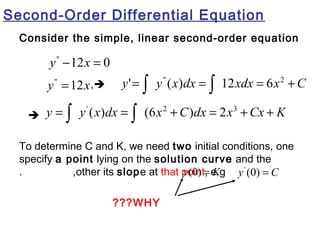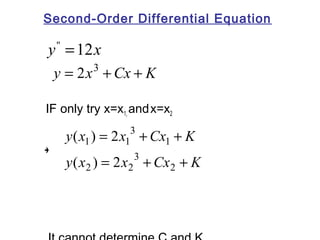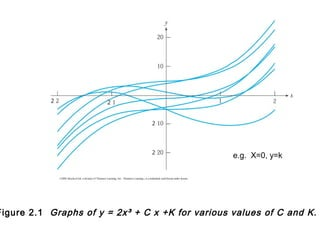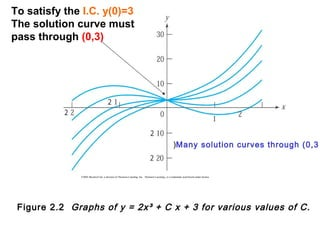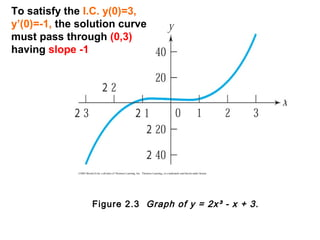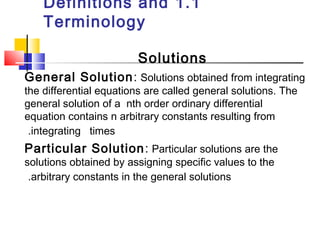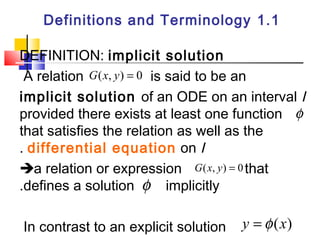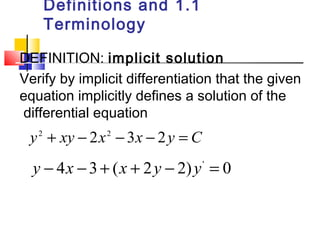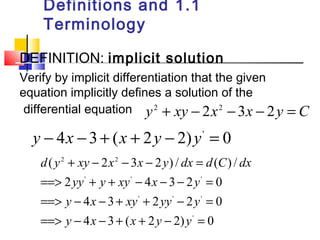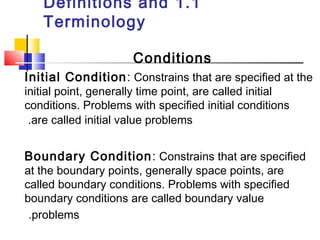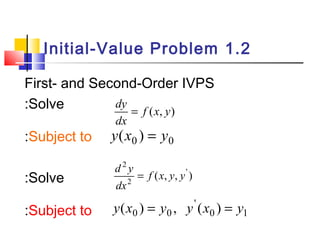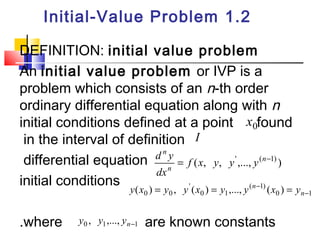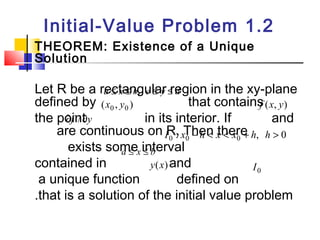1 sur 39

### Persamaan Differensial Biasa 2014

• 1. Ordinary Differential Equations
• 2. CHAPTER 1 Introduction to Differential Equations 1.1 Definitions and Terminology 1.2 Initial-Value Problems 1.3 Differential Equation as Mathematical Models
• 3. Where do ODE’s arise All branches of Engineering- Economics- Biology and Medicine- Chemistry, Physics etc- Anytime you wish to find out how something changes with time (and (sometimes space
• 4. Definitions and 1.1 Terminology DEFINITION: differential equation An equation containing the derivative of one or more dependent variables, with respect to one or more independent variables is said to be a differential . ( equation (DE
• 5. Definitions and 1.1 Terminology Recall Calculus Definition of a Derivative If , the derivative of or With respect to is defined as y = f (x) y f (x) x f x h f x = + - lim ( ) ( ) h dy dx h 0 ® y' , df f ' (x) The derivative is also denoted by or dx
• 6. Definitions and 1.1 Terminology Recall the Exponential function y = f (x) = e2x dependent variable: y independent variable: x dy x x e y e d x dx d e = = é dx dx ( 2 x ) 2 (2 ) ù 2 2 2 = = úû êë
• 7. Definitions and 1.1 Terminology Differential Equation: Equations that involve dependent variables and their derivatives with respect to the independent variables. Differential Equations are classified by type, order and linearity .
• 8. Definitions and 1.1 Terminology Differential Equations are classified by type, order and linearity . TYPE There are two main types of differential equation: . ” “ordinary” and “partial
• 9. Definitions and 1.1 Terminology Ordinary differential equation (ODE( Differential equations that involve only ONE independent variable are called ordinary differential equations. Examples: dy 2 + 5 = - + 6 y = 0 2 dx + dy = 2 + d y dy x y , , and y ex dx dx dx dt dt only ordinary (or total ( derivatives
• 10. Definitions and 1.1 Terminology Partial differential equation (PDE( Differential equations that involve two or more independent variables are called partial differential equations. Examples: u ¶ and u t 2 2 ¶ 2 2 t u x u - ¶ ¶ = ¶ ¶ ¶ 2 = - ¶ only partial derivatives v x y ¶ ¶
• 11. Definitions and 1.1 Terminology ORDER The order of a differential equation is the order of the highest derivative found in .the DE 3 2 d y - = ÷ø y ex dy + 5æ 4 ö dx çè dx 2 second order first order
• 12. Definitions and 1.1 Terminology xy' - y2 = ex :Written in differential form M(x, y)dx + N(x, y)dy = 0 y'' = x3 first order F(x, y, y' ) = 0 second order F(x, y, y' , y'' ) = 0
• 13. Definitions and 1.1 Terminology LINEAR or NONLINEAR An n-th order differential equation is said to be linear if the function is linear in the variables  F(x, y, y' ,......y(n) ) = 0 y, y' ,... y(n-1) - 1 a x dy n n a x d y n a x d y 1 a x y g x n + + + + = - ( ) ( ) 1 ... 1( ) 0 ( ) ( ) dx dx dx n n - there are no multiplications among dependent variables and their derivatives. All coefficients are functions of independent variables. A nonlinear ODE is one that is not linear, i.e. does not .have the above form
• 14. Definitions and 1.1 Terminology LINEAR or NONLINEAR x dy 4 + ( y - x) = 0 dx ( y - x)dx + 4xdy = 0 or linear first-order ordinary differential equation y'' - 2y' + y = 0 linear second-order ordinary differential equation 3 d y + 3 - 5 = 3 y ex x dy dx dx  linear third-order ordinary differential equation
• 15. Definitions and 1.1 Terminology LINEAR or NONLINEAR (1- y) y' + 2y = ex coefficient depends on y nonlinear first-order ordinary differential equation 2 + y = dx d y sin( ) 0 2 nonlinear function of y nonlinear second-order ordinary differential equation 4 + y = dx 2 0 d y power not 1 4  nonlinear fourth-order ordinary differential equation
• 16. Definitions and 1.1 Terminology LINEAR or NONLINEAR :NOTE ... 3! 5! 7! sin( ) 3 5 7 y = y - y + y - y + -¥ < x < ¥ ... 2! 4! 6! cos( ) 1 2 4 6 y = - y + y - y + -¥ < x < ¥
• 17. Definitions and 1.1 Terminology Solutions of ODEs DEFINITION: solution of an ODE Any function f , defined on an interval I and possessing at least n derivatives that are continuous on I, which when substituted into an n-th order ODE reduces the equation to an identity, is said to be a . solution of the equation on the interval
• 18. Definitions and 1.1 Terminology Namely, a solution of an n-th order ODE is a function which possesses at least n derivatives and for which for F(all x, x f in (x), I f ' (x), f (n) (x)) = 0 We say that satisfies the differential equation .on I
• 19. Definitions and 1.1 Terminology Verification of a solution by substitution :Example y'' - 2y' + y = 0 ; y = xex  y' = xex + ex , y'' = xex + 2ex :left hand side y'' - 2y' + y = (xex + 2ex ) - 2(xex + ex ) + xex = 0 right-hand side: 0 The DE possesses the constant y=0 trivial solution
• 20. Definitions and 1.1 Terminology DEFINITION: solution curve A graph of the solution of an ODE is called a solution curve, or an integral curve of .the equation
• 21. Definitions and Terminology 1.1 DEFINITION: families of solutions A solution containing an arbitrary constant (parameter) represents a set G(x, y,c) = 0 of solutions to an ODE called a one-parameter . family of solutions A solution to an n−th order ODE is a n-parameter family of F(x, y, y' ,......y(n) ) = 0 . solutions Since the parameter can be assigned an infinite number of values, an ODE can have an infinite .number of solutions
• 22. Definitions and 1.1 Terminology Verification of a solution by substitution :Example y' + y = 2 φ(x) = 2 + ke- x y' + y = 2 φ(x) = 2 + ke-x φ' (x) = -ke- x φ' (x) + φ(x) = -ke- x + 2 + ke- x = 2 
• 23. ©2003 Brooks/Cole, a division of Thomson Learning, Inc. Thomson Learning™ is a trademark used herein under license. y = 2 + ke-x Figure 1.1 Integral curves of y’ + y = 2 for k = 0, 3, –3, 6, and –6.
• 24. Definitions and 1.1 Terminology Verification of a solution by substitution :Example  for all ,  y y ' = +1 x φ(x) = x ln(x) + Cx x > 0 φ' (x) = ln(x) +1+C φ' ( ) = ln( ) + +1 = φ( x ) +1 x x x x Cx x
• 25. ©2003 Brooks/Cole, a division of Thomson Learning, Inc. Thomson Learning™ is a trademark used herein under license. 1 (xe e c) x y = x - x + Figure 1.2 Integral curves of y’ + ¹ y = ex for c =0,5,20, -6, and –10. x
• 26. Second-Order Differential Equation : Example is a solution of :By substitution φ(x) = 6cos(4x) -17sin(4x) y'' +16x = 0 x x = - - φ ' 24sin(4 ) 68cos(4 ) = - + φ '' 96cos(4 ) 272sin(4 ) φ '' 16φ 0 + = x x F(x, y, y' , y'' ) = 0 F( x,φ(x),φ' (x),φ(x)'' ) = 0
• 27. Second-Order Differential Equation Consider the simple, linear second-order equation y'' -12x = 0 y'' =12x , y ' = ò y '' ( x ) dx = ò 12 xdx = 6 x 2 +C y = ò y ' ( x ) dx = ò (6 x 2 +C ) dx = 2 x 3 +Cx + K  To determine C and K, we need two initial conditions, one specify a point lying on the solution curve and the . ,other its slope at that point, e.g ???WHY y(0) = K y' (0) = C
• 28. Second-Order Differential Equation y'' =12x y = 2x3 +Cx + K IF only try x=x1, and x=x2  3 y x = x + Cx + K 3 1 ( ) 2 1 1 ( ) 2 y x = x + Cx + K 2 2 2 ,It cannot determine C and K
• 29. ©2003 Brooks/Cole, a division of Thomson Learning, Inc. Thomson Learning™ is a trademark used herein under license. e.g. X=0, y=k Figure 2.1 Graphs of y = 2x³ + C x +K for various values of C and K.
• 30. To satisfy the I.C. y(0)=3 The solution curve must pass through (0,3) Many solution ) curves through (0,3 ©2003 Brooks/Cole, a division of Thomson Learning, Inc. Thomson Learning™ is a trademark used herein under license. Figure 2.2 Graphs of y = 2x³ + C x + 3 for various values of C.
• 31. To satisfy the I.C. y(0)=3, y’(0)=-1, the solution curve must pass through (0,3) having slope -1 ©2003 Brooks/Cole, a division of Thomson Learning, Inc. Thomson Learning™ is a trademark used herein under license. Figure 2.3 Graph of y = 2x³ - x + 3.
• 32. Definitions and 1.1 Terminology Solutions General Solution: Solutions obtained from integrating the differential equations are called general solutions. The general solution of a nth order ordinary differential equation contains n arbitrary constants resulting from .integrating times Particular Solution: Particular solutions are the solutions obtained by assigning specific values to the .arbitrary constants in the general solutions
• 33. Definitions and Terminology 1.1 DEFINITION: implicit solution A relation G(x, y) = 0 is said to be an implicit solution of an ODE on an interval I provided there exists at least one function that satisfies the relation as well as the . differential equation on I a relation or expression that .defines a solution implicitly In contrast to an explicit solution y =f (x) f f G(x, y) = 0
• 34. Definitions and 1.1 Terminology DEFINITION: implicit solution Verify by implicit differentiation that the given equation implicitly defines a solution of the differential equation y2 + xy - 2x2 - 3x - 2y = C y - 4x - 3 + (x + 2y - 2) y' = 0
• 35. Definitions and 1.1 Terminology DEFINITION: implicit solution Verify by implicit differentiation that the given equation implicitly defines a solution of the differential equation y2 + xy - 2x2 - 3x - 2y = C y - 4x - 3 + (x + 2y - 2) y' = 0 d ( y + xy - 2 x - 3 x - 2 y ) / dx = d ( C ) / dx yy y xy x y ==> + + - - - = 2 ' ' 4 3 2 ' 0 y x xy yy y ==> - - + + - = 4 3 ' 2 ' 2 ' 0 y x x y y 4 3 ( 2 2) ' 0 2 2 ==> - - + + - =
• 36. Definitions and 1.1 Terminology Conditions Initial Condition: Constrains that are specified at the initial point, generally time point, are called initial conditions. Problems with specified initial conditions .are called initial value problems Boundary Condition: Constrains that are specified at the boundary points, generally space points, are called boundary conditions. Problems with specified boundary conditions are called boundary value .problems
• 37. Initial-Value Problem 1.2 First- and Second-Order IVPS :Solve :Subject to :Solve :Subject to f (x, y) dy = dx y(x0 ) = y0 ( , , ' ) d y = 2 2 f x y y dx 0 1 ' y(x0 ) = y0 , y (x ) = y
• 38. Initial-Value Problem 1.2 DEFINITION: initial value problem An initial value problem or IVP is a problem which consists of an n-th order ordinary differential equation along with n initial conditions defined at a point x0 found in the interval of definition I differential equation d n y = f ( x , y , y ' ,..., y (n-1) ) n initial conditions dx y x y y x y y n x y = = - = n .where are known constants 0 1 ( 1) 0 1 ' ( 0 ) 0 , ( ) ,..., ( ) - y0 , y1,..., yn-1
• 39. Initial-Value Problem 1.2 THEOREM: Existence of a Unique Solution Let R be a rectangular a £ x £ b, c £ y £ region d in the xy-plane defined by (x0 , y0 ) that contains f (x, y) the point ¶f / ¶y in its interior. If and are continuous on R, Then there exists some interval I0 : x0 - h < x < x0 + h, h > 0 a £ x £ b contained in y(x) and I0 a unique function defined on .that is a solution of the initial value problem